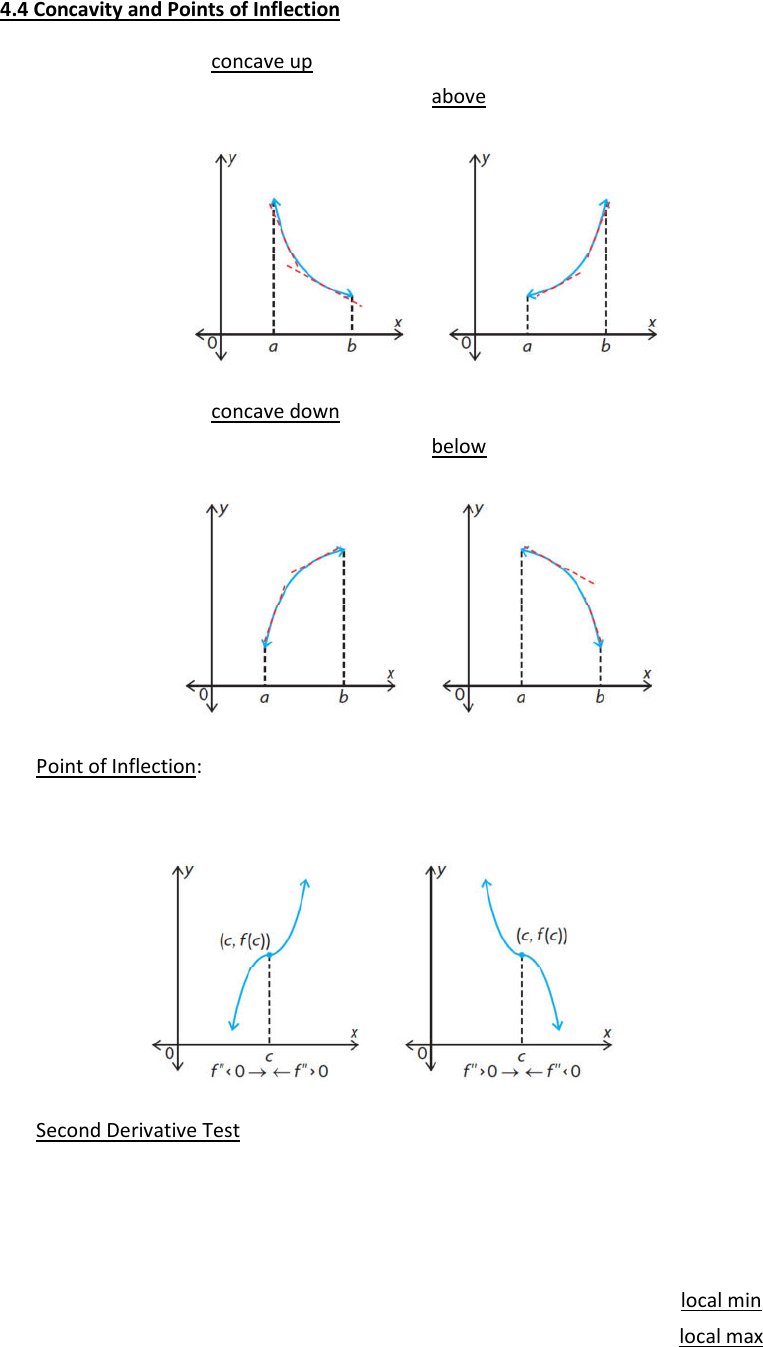# MAT135H1 Lecture Notes - Lecture 3: Inflection, If And Only If

24 views3 pages4.4 Concavity and Points of Inflection
A function
()fx
is concave up on an interval if
''( ) 0fx>
o The graph of the function is above the tangent on every point of the interval
A function
()fx
is concave down on an interval if
''( ) 0fx<
o The graph of the function is below the tangent on every point of the interval
Point of Inflection:
o a point on a graph where
()fx
changes from concave up to concave down and vice versa
o a point of inflection occurs when
''( ) 0fx=
or undefined
Second Derivative Test:
o another test used to find local max or min values
! find critical points using
'( ) 0fx=
! find
''( )fx
if it exists
! evaluate
''( )fx
at critical points
if
''( ) 0fc>
, then the critical point is a local min
if
''( ) 0fc<
, then the critical point is a local max
if
''( ) 0fc=
, then the test fails; use the first derivative test
Unlock document

This preview shows page 1 of the document.
Unlock all 3 pages and 3 million more documents.